# Tutorial for R Package match2C

options(scipen = 99)
options(digits = 3)
library(match2C)
library(ggplot2)

# Introduction

## Preparation of data

This file serves as an introduction to the R package match2C. We first load the package and an illustrative dataset from Rouse (1995). For the purpose of illustration, we will mostly work with 6 covariates: two nominal (black and female), two ordinal (father’s education and mother’s education), and two continuous (family income and test score). Treatment is an instrumental-variable-defined exposure, equal to $$1$$ if the subject is doubly encouraged, meaning the both the excess travel time and excess four-year college tuition are larger than the median, and to be $$0$$ if the subject is doubly discouraged. There are $$1,122$$ subjects that are doubly encouraged (treated), and $$1,915$$ that are doubly discouraged (control).

Below, we specify covariates to be matched (X) and the exposure (Z), and fit a propensity score model.

attach(dt_Rouse)
X = cbind(female,black,bytest,dadeduc,momeduc,fincome) # covariates to be matched
Z = IV # IV-defined exposure in this dataset

# Fit a propensity score model
family=binomial)$fitted.values # Number of treated and control n_t = sum(Z) # 1,122 treated n_c = length(Z) - n_t # 1,915 control dt_Rouse$propensity = propensity
detach(dt_Rouse)

## Glossary of Matching Terms

We define some useful statistical matching terminologies:

• Bipartite Matching: Matching control subjects to treated subjects based on a binary treatment status.

• Tripartite Matching: Matching control subjects to treated subjects based on a tripartite network. A tripartite network consists of two bipartite networks: a left network and a right network, where the right network is a mirror copy of the left network in nodes, but with possibly different distance structure. Typically the left network is responsible for close pairing and the right network is responsible for balancing; See Zhang et al., 2021 for details.

• Pair Matching: Matching one control subject to one treated subject.

• Optimal Matching: Matching control subjects to treated subjects such that some properly defined sum of total distances is minimized.

• Propensity Score: The propensity score is the conditional probability of assignment to a particular treatment given a vector of observed covariates (Rosenbaum and Rubin, 1983).

• Mahalanobis Distance: A multivariate measure of covariate distance between units in a sample (Mahalanobis, 1936). The squared Mahalanobis distance is equal to the difference in covariate values of treated units and matched control units, divided by the covariate’s standard deviation. Mahalanobis distance takes into account the correlation structure among covariates. The distance is zero if two units have the same value for all covariates and increases as two units become more dissimilar.

• Exact Matching: Matching cases to controls requiring the same value of a nominal covariate.

• Fine Balance: A matching technique that balances exactly the marginal distribution of one nominal variable or the joint distribution of several nominal variables in the treated and control groups after matching (Rosenbaum et al., 2007; Yu et al., 2020).

For more details on statistical matching and statistical inference procedures after matching, see Observational Studies (Rosenbaum, 2002) and Design of Observational Studies (Rosenbaum, 2010).

# Statistical Matching Workflow: Match, Check Blance, and (Possibly) Iterate

## An Overview of the Family of Three Matching Functions match_2C, match_2C_mat, and match_2C_list

In the package match2C, three functions are primarily responsible for the main task statistical matching. These three functions are match_2C, match_2C_mat, and match_2C_list. We will examine more closely their differences and illustrate their usage with numerous examples in later sections. In this section we give a high-level outline of what each of them does. In short, the three functions have the same output format (details in the next section), but are different in their inputs.

Function match_2C_mat takes as input at least one distance matrix. A distance matrix is a n_t-by-b_c matrix whose ij-th entry encodes a measure of distance (or similarity) between the i-th treated and the j-th control subject. Hence, function match_2C_mat is most handy for users who are familiar with constructing and working with distance matrices. One commonly-used way to construct a distance matrix is to use the function match_on in the package optmatch (Hansen, 2007).

Function match_2C_list is similar to match_2C_mat except that it requires at least one distance list as input. A list representation of a treatment-by-control distance matrix consists of the following arguments:

• start_n: a vector containing the node numbers of the start nodes of each arc in the network.
• end_n: a vector containing the node numbers of the end nodes of each arc in the network.
• d: a vector containing the integer cost of each arc in the network.

Nodes 1,2,…,n_t correspond to n_t treatment nodes, and n_t + 1, n_t + 2, …, n_t + n_c correspond to n_c control nodes. Note that start_n, end_n, and d have the same lengths, all of which equal to the number of edges. Functions create_list_from_scratch and create_list_from_mat in the package allow users to construct a (possibly sparse) distance list with a possibly user-specified distance measure. We will discuss how to construct distance lists in later sections.

Function match_2C is a wrap-up of match_2C_list with pre-specified distance list structures. For the left network, a Mahalanobis distance between covariates X is adopted; For the right network, an L-1 distance between the propensity score is used. A large penalty is applied so that the algorithm prioritizes balancing the propensity score distributions in the treated and matched control groups, followed by minimizing the sum of within-matched-pair Mahalanobis distances. Function match_2C further allows fine-balancing the joint distribution of a few key covariates. The hierarchy goes in the order of fine-balance >> propensity score distribution >> within-pair Mahalanobis distance.

## Object Returned by match_2C, match_2C_mat, and match_2C_list

Objects returned by the family of matching functions match_2C, match_2C_mat, and match_2C_list are the same in format: a list of the following three elements:

• feasible: 0/1 depending on the feasibility of the matching problem;

• data_with_matched_set_ind: a data frame that is the same as the original data frame, except that a column called matched_set and a column called distance are added to it. Variable matched_set assigns 1,2,…,n_t to each matched set, and NA to controls not matched to any treated. Variable distance records the control-to-treated distance in each matched pair, and assigns NA to all treated and controls that are left unmatched. If matching is not feasible, NULL will be returned;

• matched_data_in_order: a data frame organized in the order of matched sets and otherwise the same as data_with_matched_set_ind. Null will be returned if the matching is unfeasible.

Let’s take a look at an example output returned by the function match_2C_list. The matching problem is indeed feasible:

# Check feasibility
matching_output_example$feasible #>  1 Let’s take a look at the data frame data_with_matched_set_ind. Note that it is indeed the same as the original dataset except that a column matched_set and a column distance are appended. Observe that the first six instances belong to $$6$$ different matched sets; therefore matched_set is from $$1$$ to $$6$$. The first six instances are all treated subjects so distance is NA. # Check the original dataset with two new columns head(matching_output_example$data_with_matched_set_ind, 6)
#> 1     12     1      1     1        0   40.6       0       0       0       0
#> 2     14     1      1     0        0   46.3       0       0       0       0
#> 3     12     1      1     0        0   60.4       0       0       0       0
#> 4     14     1      1     0        0   60.9       0       0       1       0
#> 5     16     1      1     0        0   45.8       0       0       0       0
#> 6     12     1      0     0        0   60.2       0       1       0       0
#> 1    9500        0  1          0          0       0       0             1
#> 2   18000        0  1          0          0       0       0             1
#> 3   22500        0  1          1          0       1       0             4
#> 4   22500        0  1          0          0       0       2             4
#> 5       0        1  1          1          0       1       0             1
#> 6   62000        0  1          0          0       3       0             4
#>   income_quartile propensity matched_set distance
#> 1               1      0.416           1       NA
#> 2               2      0.408           2       NA
#> 3               3      0.353           3       NA
#> 4               3      0.347           4       NA
#> 5               0      0.428           5       NA
#> 6               4      0.305           6       NA

Finally, matched_data_in_order is data_with_matched_set_ind organized in the order of matched sets. Note that the first $$2$$ subjects belong to the same matched set; the next two subjects belong to the second matched set, and etc.

# Check dataframe organized in matched set indices
head(matching_output_examplematched_data_in_order, 6) #> educ86 twoyr female black hispanic bytest dadsome dadcoll momsome momcoll #> 1 12 1 1 1 0 40.6 0 0 0 0 #> 397 13 1 1 1 0 42.7 0 0 0 0 #> 2 14 1 1 0 0 46.3 0 0 0 0 #> 565 12 1 1 0 0 45.7 0 0 0 0 #> 3 12 1 1 0 0 60.4 0 0 0 0 #> 1312 15 0 1 0 0 58.6 1 0 0 0 #> fincome fincmiss IV dadneither momneither dadeduc momeduc test_quartile #> 1 9500 0 1 0 0 0 0 1 #> 397 3500 0 0 1 0 1 0 1 #> 2 18000 0 1 0 0 0 0 1 #> 565 22500 0 0 0 0 0 0 1 #> 3 22500 0 1 1 0 1 0 4 #> 1312 31500 0 0 0 0 2 0 3 #> income_quartile propensity matched_set distance #> 1 1 0.416 1 NA #> 397 1 0.413 1 1.998 #> 2 2 0.408 2 NA #> 565 3 0.406 2 0.361 #> 3 3 0.353 3 NA #> 1312 3 0.350 3 0.852 ## Checking Balance Statistical matching belongs to the design stage of an observational study. The ultimate goal of statistical matching is to embed observational data into an approximate randomized controlled trial and the matching process should always be conducted without access to the outcome data. Not looking at the outcome at the design stage means researchers could in principle keep adjusting their matched design until some pre-specified design goal is achieved. A rule of thumb is that the standardized differences of each covariate, i.e., difference in means after matching divided by pooled standard error before matching, is less than 0.1. Function check_balance in the package provides simple balance check and visualization. In the code chunk below, matching_output_example is an object returned by the family of matching functions match_2C_list/match_2C/match_2C_mat (we give details on how to use these functions later). Function check_balance then takes as input a vector of treatment status Z, an object returned by match_2C (or match_2C_mat or match_2C_list), a vector of covariate names for which we would like to check balance, and output a balance table. There are six columns of the balance table: 1. Mean covariate values in the treated group (Z = 1) before matching. 2. Mean covariate values in the control group (Z = 0) before matching. 3. Standardized differences before matching. 4. Mean covariate values in the treated group (Z = 1) after matching. 5. Mean covariate values in the control group (Z = 0) after matching. 6. Standardized differences after matching. tb_example = check_balance(Z, matching_output_example, cov_list = c('female', 'black', 'bytest', 'fincome', 'dadeduc', 'momeduc', 'propensity'), plot_propens = FALSE) print(tb_example) #> Z = 1 Z = 0 (Bef) Std. Diff (Bef) Z = 0 (Aft) Std. Diff (Aft) #> female 0.580 0.558 0.0321 0.580 0.00000 #> black 0.191 0.185 0.0106 0.181 0.01774 #> bytest 51.884 53.040 -0.0976 52.032 -0.01251 #> fincome 21630.125 23439.164 -0.0722 21479.501 0.00601 #> dadeduc 1.102 1.171 -0.0409 1.111 -0.00527 #> momeduc 0.935 0.996 -0.0375 0.912 0.01427 #> propensity 0.373 0.367 0.1146 0.373 0.00264 Function check_balance may also plot the distribution of the propensity score among the treated subjects, all conrol subjects, and the matched control subjects by setting option plot_propens = TRUE and supplying the option propens with estimated propensity scores as shown below. In the figure below, the blue curve corresponds to the propensity score distribution among 1,122 treated subjects, the red curve among 1,915 control subjects, and the green curve among 1,122 matched controls. It is evident that after matching, the propensity score distribution aligns better with that of the treated subjects. tb_example = check_balance(Z, matching_output_example, cov_list = c('female', 'black', 'bytest', 'fincome', 'dadeduc', 'momeduc', 'propensity'), plot_propens = TRUE, propens = propensity)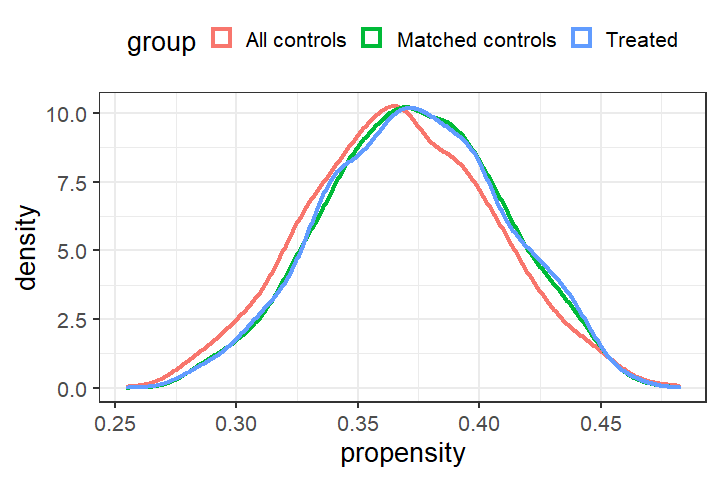# Introducing the Main Function match_2C ## A Basic Match with Minimal Input Function match_2C is a wrapper function of match_2C_list with a carefully-chosen distance structure. Compare to match_2C_list and match_2C_mat, match_2C is less flexible; however, it requires minimal input from the users’ side, works well in most cases, and therefore is of primary interest to most users. The minimal input to function match_2C is the following: 1. treatment indicator vector, 2. a matrix of covariates to be matched, 3. a vector of estimated propensity score, and 4. the original dataset to which match sets information is attached. By default, match_2C performs a statistical matching that: 1. maximally balances the marginal distribution of the propensity score in the treated and matched control group, and 2. subject to 1, minimizes the within-matched-pair Mahalanobis distances. The code chunk below displays how to perform a basic match using function match_2C with minimal input, and then check the balance of such a match. The balance is very good and the propensity score distributions in the treated and matched control group almost perfectly align with each other. # Perform a matching with minimal input matching_output = match_2C(Z = Z, X = X, method = 'robust maha', propensity = propensity, dataset = dt_Rouse) tb = check_balance(Z, matching_output, cov_list = c('female', 'black', 'bytest', 'fincome', 'dadeduc', 'momeduc', 'propensity'), plot_propens = TRUE, propens = propensity)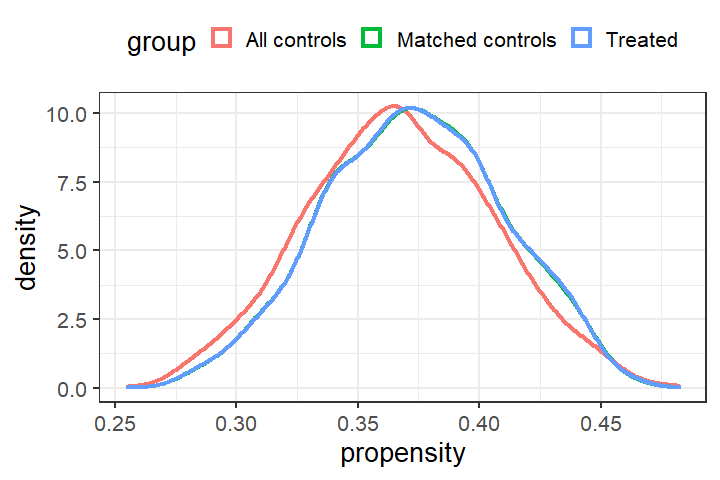print(tb) #> Z = 1 Z = 0 (Bef) Std. Diff (Bef) Z = 0 (Aft) Std. Diff (Aft) #> female 0.580 0.558 0.0321 0.580 0.000000 #> black 0.191 0.185 0.0106 0.191 0.000000 #> bytest 51.884 53.040 -0.0976 51.904 -0.001750 #> fincome 21630.125 23439.164 -0.0722 21579.323 0.002027 #> dadeduc 1.102 1.171 -0.0409 1.121 -0.011603 #> momeduc 0.935 0.996 -0.0375 0.930 0.002745 #> propensity 0.373 0.367 0.1146 0.373 0.000421 ## Incorporating Exact Matching Constraints Researchers can also incorporate the exact matching constraints by specifying the variables to be exactly matched in the option exact. In the example below, we match exactly on father’s education and mother’s education. The matching algorithm still tries to find a match that maximally balance the propensity score distribution, and then minimzies the treated-to-control total distances, subject to the exact matching constraints. One can check that father’s education and mother’s education are exactly matched. Moreover, since the matching algorithm separates balancing the propensity score from exact matching, the propensity score distributions are still well balanced. # Perform a matching with minimal input matching_output_with_exact = match_2C(Z = Z, X = X, exact = c('dadeduc', 'momeduc'), propensity = propensity, dataset = dt_Rouse) # Check exact matching head(matching_output_with_exactmatched_data_in_order[, c('female', 'black', 'bytest',
'propensity', 'IV', 'matched_set')])
#>      female black bytest fincome dadeduc momeduc propensity IV matched_set
#> 1         1     1   40.6    9500       0       0      0.416  1           1
#> 52        1     1   45.0   18000       0       0      0.391  0           1
#> 2         1     0   46.3   18000       0       0      0.408  1           2
#> 2547      1     0   45.4   14000       0       0      0.416  0           2
#> 3         1     0   60.4   22500       1       0      0.353  1           3
#> 417       1     0   56.7   22500       1       0      0.366  0           3

# Check overall balance
tb = check_balance(Z, matching_output_with_exact,
cov_list = c('female', 'black', 'bytest', 'fincome', 'dadeduc', 'momeduc', 'propensity'),
plot_propens = TRUE, propens = propensity)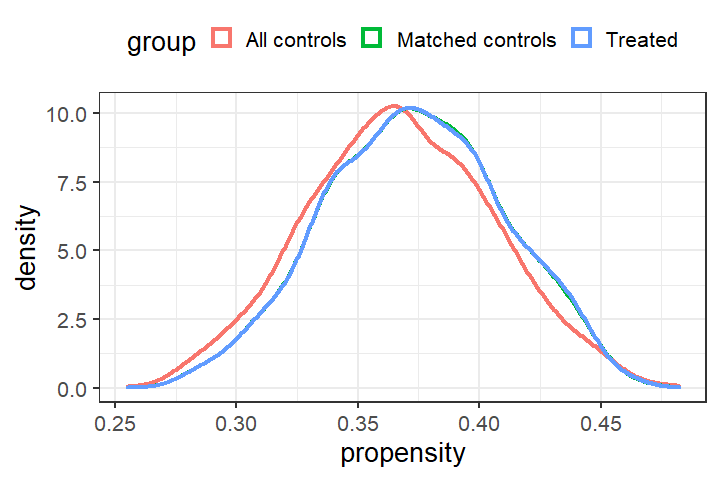## Incorporating Fine Balancing Constraints

Function match_2C also allows incorporating the (near-)fine balancing constraints. (Near-)fine balance refers to maximally balancing the marginal distribution of a nominal variable, or more generally the joint distribution of a few nominal variables, in the treated and matched control groups. Option fb in the function match_2C serves this purpose. Once the fine balance is turned on, match_2C then performs a statistical matching that:

1. maximally balances the marginal distribution of nominal levels specified in the option fb,
2. subject to 1. maximally balances the marginal distribution of the propensity score in the treated and matched control group, and
3. subject to 2, minimizes the within-matched-pair Mahalanobis distances.

The code chunk below builds upon the last match by further requiring fine balancing the nominal variable dadeduc:

# Perform a matching with fine balance
matching_output2 = match_2C(Z = Z, X = X,
propensity = propensity,
dataset = dt_Rouse,
fb_var = c('dadeduc'))

We examine the balance and the variable dadeduc is indeed finely balanced.

# Perform a matching with fine balance
tb2 = check_balance(Z, matching_output2,
cov_list = c('female', 'black', 'bytest', 'fincome', 'dadeduc', 'momeduc', 'propensity'),
plot_propens = TRUE, propens = propensity)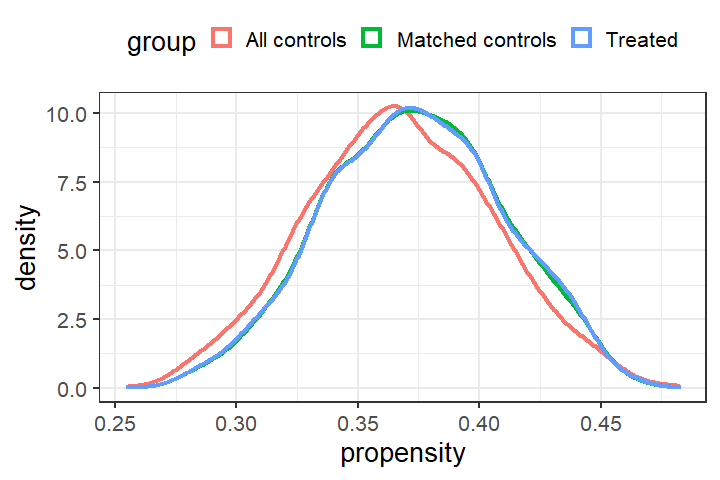print(tb2)
#>                Z = 1 Z = 0 (Bef) Std. Diff (Bef) Z = 0 (Aft) Std. Diff (Aft)
#> female         0.580       0.558          0.0321       0.580        0.000000
#> black          0.191       0.185          0.0106       0.191        0.000000
#> bytest        51.884      53.040         -0.0976      51.946       -0.005288
#> fincome    21630.125   23439.164         -0.0722   21441.622        0.007521
#> dadeduc        1.102       1.171         -0.0409       1.102        0.000000
#> momeduc        0.935       0.996         -0.0375       0.929        0.003843
#> propensity     0.373       0.367          0.1146       0.373        0.000658

One can further finely balance the joint distribution of multiple nominal variables. The code chunk below finely balances the joint distribution of father’s (4 levels) and mother’s (4 levels) education ($$4 \times 4 = 16$$ levels in total).

# Perform a matching with fine balance on dadeduc and moneduc
matching_output3 = match_2C(Z = Z, X = X,
propensity = propensity,
dataset = dt_Rouse,
tb3 = check_balance(Z, matching_output2,
cov_list = c('female', 'black', 'bytest', 'fincome', 'dadeduc', 'momeduc', 'propensity'),
plot_propens = FALSE)
print(tb3)
#>                Z = 1 Z = 0 (Bef) Std. Diff (Bef) Z = 0 (Aft) Std. Diff (Aft)
#> female         0.580       0.558          0.0321       0.580        0.000000
#> black          0.191       0.185          0.0106       0.191        0.000000
#> bytest        51.884      53.040         -0.0976      51.946       -0.005288
#> fincome    21630.125   23439.164         -0.0722   21441.622        0.007521
#> dadeduc        1.102       1.171         -0.0409       1.102        0.000000
#> momeduc        0.935       0.996         -0.0375       0.929        0.003843
#> propensity     0.373       0.367          0.1146       0.373        0.000658

## Sparsifying the Network to Match Faster and Match Bigger Datasets

Sparsifying a network refers to deleting certain edges in a network. Edges deleted typically connect a treated and a control subject that are unlikely to be a good match. Using the estimated propensity score as a caliper to delete unlikely edges is the most commonly used strategy. For instance, a propensity score caliper of 0.05 would result in deleting all edges connecting one treated and one control subject whose estimated propensity score differs by more than 0.05. Sparsifying the network has potential to greatly facilitate computation (Yu et al., 2020).

Function match_2C allows users to specify two caliper sizes on the propensity scores, caliper_left for the left network and caliper_right for the right network. If users are interested in specifying a caliper other than the propensity score and/or specifying an asymmetric caliper (Yu and Rosenbaum, 2020), functions match_2C_list serves this purpose (see Section 4 for details). Moreover, users may further trim the number of edges using the option k_left and k_right. By default, each treated subject in the network is connected to each of the n_c control subjects. Option k_left allows users to specify that each treated subject gets connected only to the k_left control subjects who are closest to the treated subject in the propensity score in the left network. For instance, setting k_left = 200 results in each treated subject being connected to at most 200 control subjects closest in the propensity score in the left network. Similarly, option k_right allows each treated subject to be connected to the closest k_right controls in the right network. Options caliper_low, caliper_high, k_left, and k_right can be used together.

Below, we give a simple example illustrating the usage of caliper and contrasting the running time of applying match_2C without any caliper, one caliper on the left, and both calipers on the left and the right. Using double calipers in this case roughly cuts the computation time by almost two-thirds.

# Timing the vanilla match2C function
ptm <- proc.time()
matching_output2 = match_2C(Z = Z, X = X,
propensity = propensity,
dataset = dt_Rouse)
time_vanilla = proc.time() - ptm

# Timing the match2C function with caliper on the left
ptm <- proc.time()
matching_output_one_caliper = match_2C(Z = Z, X = X, propensity = propensity,
caliper_left = 0.05, caliper_right = 0.05,
k_left = 100,
dataset = dt_Rouse)
time_one_caliper = proc.time() - ptm

# Timing the match2C function with caliper on the left and right
ptm <- proc.time()
matching_output_double_calipers = match_2C(Z = Z, X = X,
propensity = propensity,
caliper_left = 0.05, caliper_right = 0.05,
k_left = 100, k_right = 100,
dataset = dt_Rouse)
time_double_caliper = proc.time() - ptm

rbind(time_vanilla, time_one_caliper, time_double_caliper)[,1:3]
#>                     user.self sys.self elapsed
#> time_vanilla             6.91     0.43    7.39
#> time_one_caliper         5.00     0.28    5.31
#> time_double_caliper      2.45     0.03    2.50

Caveat: if caliper sizes are too small, the matching may be unfeasible. See the example below. In such an eventuality, users are advised to increase the caliper size and/or remove the exact matching constraints.

# Perform a matching with fine balance on dadeduc and moneduc
matching_output_unfeas = match_2C(Z = Z, X = X, propensity = propensity,
dataset = dt_Rouse,
caliper_left = 0.001)
#> Hard caliper fails. Please specify a soft caliper.
#> Matching is unfeasible. Please increase the caliper size or remove
#>         the exact matching constraints.

## Force including certain controls into the matched cohort

Sometimes, researchers might want to include certain controls in the final matched cohort. Option include in the function match_2C serves this purpose. The option include is a binary vectors (0’s and 1’s) whose length equal to the total number of controls, with 1 in the i-th entry if the i-th control has to be included and 0 otherwise. For instance, the match below forces including the first 100 controls in our matched samples.


# Create a binary vector with 1's in the first 100 entries and 0 otherwise
# length(include_vec) = n_c

include_vec = c(rep(1, 100), rep(0, n_c - 100))
# Perform a matching with minimal input
matching_output_force_include = match_2C(Z = Z, X = X,
propensity = propensity,
dataset = dt_Rouse,
include = include_vec)

One can check that the first 100 controls in the original dataset are forced into the final matched samples.


matched_data = matching_output_force_include$data_with_matched_set_ind matched_data_control = matched_data[matched_data$IV == 0,]
head(matched_data_control) # Check the matched_set column
#> 20     14     1      1     1        0   44.9       0       0       0       0
#> 21     12     1      1     1        0   60.3       0       0       0       0
#> 22     14     1      0     1        0   48.3       0       0       0       0
#> 23     12     1      0     1        0   45.8       0       0       0       0
#> 24     13     1      0     1        0   47.2       0       0       0       0
#> 25     13     1      0     1        0   39.3       0       0       0       0
#> 20    9500        0  0          1          0       1       0             1
#> 21       0        1  0          1          1       1       1             4
#> 22       0        1  0          0          0       0       0             2
#> 23    9500        0  0          0          0       0       0             1
#> 24    3500        0  0          1          0       1       0             2
#> 25   62000        0  0          1          0       1       0             1
#>    income_quartile propensity matched_set distance
#> 20               1      0.400         848     1.77
#> 21               0      0.351         765     3.67
#> 22               0      0.385         974     1.41
#> 23               1      0.384         797     0.91
#> 24               1      0.385         251     1.78
#> 25               4      0.357         549     5.55

# Function match_2C_mat: Matching with Two User-Supplied Distance Matrices

## An Overview

We illustrate how to use the function match_2C_mat in this section. The function match_2C_mat takes the following inputs:

• Z: A length-n vector of treatment indicator.

• X: A n-by-p matrix of covariates.

• dataset: The original dataset.

• dist_mat_1: A user-specified treatment-by-control (n_t-by-n_c) distance matrix.

• dist_mat_2: A second user-specified treatment-by-control (n_t-by-n_c) distance matrix.

• lambda: A penalty that does a trade-off between two parts of the network.

• controls: Number of controls matched to each treated. Default is 1.

• p_1: A length-n vector on which caliper_1 applies, e.g. a vector of propensity score.

• caliper_1: Size of caliper_1.

• k_1: Maximum number of controls each treated is connected to in the first network.

• p_2: A length-n vector on which caliper_2 applies, e.g. a vector of propensity score.

• caliper_2: Size of caliper_2.

• k_2: Maximum number of controls each treated is connected to in the second network.

• penalty: Penalty for violating the caliper. Set to Inf by default.

The key inputs to the function match_2C_mat are two distance matrices. The simplest way to construct a n_t-by_n_c distance matrix is to use the function match_on in the R package optmatch.

## Examples

We give two examples below to illustrate match_2C_mat.

In the first example, we construct dist_mat_1 based on the Mahalanobis distance between all covariates in X, and dist_mat_2 based on the Euclidean distance of the covariate dadeduc. A large penalty lambda is applied to the second distance matrix so that the algorithm is forced to find an optimal match that satisfies (near-)fine balance on the nominal variable dadeduc. We do not use any caliper in this example.

# Construct a distance matrix based on Mahalanobis distance
method = 'mahalanobis', data = dt_Rouse)

# Construct a second distance matrix based on variable dadeduc
dist_mat_2 = optmatch::match_on(IV ~ dadeduc, method = 'euclidean',
data = dt_Rouse)
matching_output_mat = match_2C_mat(Z, dt_Rouse, dist_mat_1, dist_mat_2,
lambda = 10000, controls = 1,
p_1 = NULL, p_2 = NULL)
#> Finish converting distance matrices to lists
#> Solving the network flow problem
#> Finish solving the network flow problem

# Examine the balance after matching
tb_mat = check_balance(Z, matching_output_mat,
cov_list = c('female', 'black', 'bytest', 'fincome', 'dadeduc', 'momeduc', 'propensity'),
plot_propens = FALSE)

print(tb_mat)
#>                Z = 1 Z = 0 (Bef) Std. Diff (Bef) Z = 0 (Aft) Std. Diff (Aft)
#> female         0.580       0.558          0.0321       0.580         0.00000
#> black          0.191       0.185          0.0106       0.191         0.00000
#> bytest        51.884      53.040         -0.0976      52.363        -0.04043
#> fincome    21630.125   23439.164         -0.0722   21538.324         0.00366
#> dadeduc        1.102       1.171         -0.0409       1.102         0.00000
#> momeduc        0.935       0.996         -0.0375       0.924         0.00659
#> propensity     0.373       0.367          0.1146       0.372         0.03109

In the second example, we further incorporate a propensity score caliper in the left network. The code chunk below implements a propensity score caliper of size 0.05 and connecting each treated only to at most 100 closest controls.

matching_output_mat_caliper = match_2C_mat(Z, dt_Rouse,
dist_mat_1, dist_mat_2,
lambda = 100000, controls = 1,
p_1 = propensity,
caliper_1 = 0.05, k_1 = 100)
#> Finish converting distance matrices to lists
#> Solving the network flow problem
#> Finish solving the network flow problem

Function match_2C_mat is meant to be of primary interest to users who are familiar with the package optmatch and constructing distance matrices using the function match_on. Package match2C offers functions that allow users to construct a distance list directly from the data, possibly with user-supplied distance functions. This is the topic of the next section.

# Function match_2C_list: Matching with Two User-Supplied Distance Lists

## Constructing Distance Lists from Data

A distance list is the most fundamental building block for network-flow-based statistical matching. Function create_list_from_mat allows users to convert a distance matrix to a distance list and function create_list_from_scratch allows users to construct a distance list directly from data. Function create_list_from_scratch is highly flexible and allows users to construct a distance list tailored to their specific needs.

The code chunk below illustrates the usage of create_list_from_mat by creating a distance list object list_0 from the distance matrix dist_mat_1. Note that the distance list has $$1,122 \times 1,915 = 2,148,630$$ edges, i.e., each of the 1,122 treated subject is connected to each of the 1,915 control subjects.

dist_mat_1 = optmatch::match_on(IV ~ female + black + bytest +
method = 'mahalanobis', data = dt_Rouse)

list_0 = create_list_from_mat(Z, dist_mat_1, p = NULL)
length(list_0$start_n) # number of edges in the network #>  2148630 identical(length(list_0$start_n), n_t*n_c) # Check # of edges is n_t * n_c
#>  TRUE

Function create_list_from_mat also allows caliper. Below, We apply a propensity score caliper of size $$0.05$$ to remove edges by setting p = propensity and caliper = 0.05. Observe that the number of edges is almost halved now.

list_1 = create_list_from_mat(Z, dist_mat_1,
p = propensity,
caliper = 0.05)
length(list_1$start_n) # Number of edges is almost halved #>  1379445 Function create_list_from_scratch allows users to construct a distance list without first creating a distance matrix. This is a great tool for users who are interested in experimenting/developing different matching strategies. Roughly speaking, create_list_from_scratch is an analogue of the function match_on in the package optmatch. Currently, there are 5 default distance specifications implemented: maha (Mahalanobis distance), L1 (L1 disance), robust maha (robust Mahalanobis distance), 0/1 (distance = 0 if and only if covariates are the same), and Hamming (Hamming distance), and other allows user-supplied distance functions. We will defer a discussion on how to use this user-supplied option to the next section. The minimal input to the function create_list_from_scratch is treatment Z and covariate matrix X. The user may choose the distance specification via the option method. Other useful options include the following: • Option exact allows users to specify variables that need to be exactly matched. • Option p allows users to specify a variable, e.g., the propensity score, as a caliper. • Options caliper_low and caliper_high set the size of this caliper. The size of the caliper is defined by [variable - caliper_low, variable + caliper_high]. Setting caliper_low and caliper_high to different magnitudes allows a so-called asymmetric caliper (Yu and Rosenbaum, 2020). If only caliper_low is used, caliper_high is then set to caliper_low by default and a symmetric caliper is used. • Option k allows users to further sparsify the network by connecting each treated only to k controls closest in the caliper. • Option penalty allows users to make the specified caliper a soft caliper, in the sense that the caliper is allowed to be violated at a cost of penalty. Option penalty is set to Inf by default, i.e., a hard caliper is implemented. Below, we give several examples below to illustrate its usage. First, we create a list representation using the Mahalanobis/Hamming/robust Mahalanobis distance without any caliper or exact matching requirement. # Mahalanobis distance on all variables dist_list_vanilla_maha = create_list_from_scratch(Z, X, exact = NULL, method = 'maha') # Hamming distance on all variables dist_list_vanilla_Hamming = create_list_from_scratch(Z, X, exact = NULL, method = 'Hamming') # Robust Mahalanobis distance on all variables dist_list_vanilla_robust_maha = create_list_from_scratch(Z, X, exact = NULL, method = 'robust maha')  We further specify a symmetric propensity score caliper of size $$0.05$$ and $$k = 100$$. # Mahalanobis distance on all variables with pscore caliper dist_list_pscore_maha = create_list_from_scratch(Z, X, exact = NULL, p = propensity, caliper_low = 0.05, k = 100, method = 'maha') # Hamming distance on all variables with pscore caliper dist_list_pscore_Hamming = create_list_from_scratch(Z, X, exact = NULL, p = propensity, caliper_low = 0.05, k = 100, method = 'Hamming') # Robust Mahalanobis distance on all variables with pscore caliper dist_list_pscore_robust_maha = create_list_from_scratch(Z, X, exact = NULL, p = propensity, caliper_low = 0.05, k = 100, method = 'robust maha')  If we specify too small a caliper, the problem may fail in the sense that some treated subjects are not connected to any control. See the example below. dist_list_pscore_maha_hard = create_list_from_scratch(Z, X, exact = NULL, p = propensity, caliper_low = 0.001, method = 'maha') #> Hard caliper fails. Please specify a soft caliper. In this case, users are advised to use a soft caliper by specifying a large penalty or increase the caliper size. See the example below. dist_list_pscore_maha_soft = create_list_from_scratch(Z, X, exact = NULL, p = propensity, caliper_low = 0.001, method = 'maha', penalty = 1000)  Next, we create a list representation without caliper; however, we insist that dad’s education is exactly matched. This can be done by setting the option exact to a vector of names of variables to be exactly matched. dist_list_exact_dadeduc_maha = create_list_from_scratch(Z, X, exact = c('dadeduc'), method = 'maha')  Finally, we create a list representation with an assymetric propensity score caliper and $$k = 100$$; moreover, we insist that both dad’s education and mom’s education are exactly matched. dist_list_exact_dad_mom_with_caliper = create_list_from_scratch(Z, X, exact = c('dadeduc', 'momeduc'), p = propensity, caliper_low = 0.05, caliper_high = 0.1, k = 100, method = 'maha')  ## Matching with One or Two Distance Lists Function match_2C_list takes as input the following arguments: • Z: A length-n vector of treatment indicator. • dataset: The original dataset. • dist_list_1: A distance list object returned by the function create_list_from_scratch. • dist_list_2: A second distance list object returned by the function create_list_from_scratch. • lambda: A penalty that controls the trade-off between two parts of the network. • controls: Number of controls matched to each treated. Default is set to 1. • overflow: A logical value indicating if overflow protection is turned on. If overflow = TRUE, then the matching is feasible as long as the left network is feasible. Default is set to FALSE. The key inputs are two distance list objects. The object dist_list_1 represents the network structure of the left network, while dist_list_2 represents the structure of the network on the right. If only one dist_list_1 is supplied (i.e., dist_list_2 = NULL), then a traditional bipartite match is performed. Option lambda is a tuning parameter that controls the relative trade-off between two networks. We give some examples below to illustrate the usage. ### Example I: Optimal Macthing within Propensity Score Caliper (Rosenbaum and Rubin, 1985) The classical methodology can be recovered using the following code. Note that in this example, we only need to construct one distance list and the match is a bipartite one. # Construct a distance list representing the network structure on the left. dist_list_pscore = create_list_from_scratch(Z, X, exact = NULL, p = propensity, caliper_low = 0.008, k = NULL, method = 'maha') # Perform matching. Set dist_list_2 = NULL as we are # performing a bipartite matching. matching_output_ex1 = match_2C_list(Z, dt_Rouse, dist_list_pscore, dist_list_2 = NULL, controls = 1) ### Example II: Optimal Macthing on the Left and Stringent Propensity Score Caliper on the Right We remove the propensity score caliper in the left network and put a more stringent one on the right. This allows the algorithm to separate close pairing (using the Mahalanobis distance on the left) and balancing (using a stringent propensity score caliper on the right). One may check that in this example, this little trick does simultaneously improve the closeness in pairing AND the overall balance. Note that we make the propensity score caliper on the right a soft caliper (by setting penalty = 100 instead of the detaul Inf) to ensure feasibility. # Mahalanobis distance on all variables; no caliper dist_list_no_caliper = create_list_from_scratch(Z, X, exact = NULL, p = NULL, method = 'maha') # Connect treated to controls within a stringent propensity score caliper. # We use a soft caliper here to ensure feasibility. dist_list_2 = create_list_from_scratch(Z = Z, X = rep(1, length(Z)), exact = NULL, p = propensity, caliper_low = 0.002, method = 'L1', k = NULL, penalty = 100) matching_output_ex2 = match_2C_list(Z, dt_Rouse, dist_list_no_caliper, dist_list_2, lambda = 1000, controls = 1) ### Example III: Exact Matching on One (or More) Variable while Balancing Others Suppose we would like to construct an optimal pair matching and insist two subjects in the same matched pair match exactly on father’s and mother’s education. We compare two implementations below. Our first implementation is a conventional one based on a bipartite graph: # Mahalanobis distance with exact matching on dadeduc and momeduc dist_list_1 = create_list_from_scratch(Z, X, exact = c('dadeduc', 'momeduc'), p = propensity, caliper_low = 0.05, method = 'maha') matching_output_ex3_1 = match_2C_list(Z, dt_Rouse, dist_list_1, dist_list_2 = NULL, lambda = NULL) Our second implementation uses the distance list on the left to ensure exact matching and the distance list on the right to balance the other covariates. # Maha distance with exact matching on dadeduc and momeduc dist_list_1 = create_list_from_scratch(Z, X, exact = c('dadeduc', 'momeduc'), method = 'maha') # Maha distance on all other variables dist_list_2 = create_list_from_scratch(Z, X[, c('female', 'black', 'bytest', 'fincome')], p = propensity, caliper_low = 0.05, method = 'maha') matching_output_ex3_2 = match_2C_list(Z, dt_Rouse, dist_list_1, dist_list_2, lambda = 100) One can easily verify that both implementations match exactly on dadeduc and momeduc; however, the second implementation achieves better overall balance. tb_ex3_1 = check_balance(Z, matching_output_ex3_1, cov_list = c('female', 'black', 'bytest', 'fincome', 'dadeduc', 'momeduc', 'propensity'), plot_propens = TRUE, propens = propensity)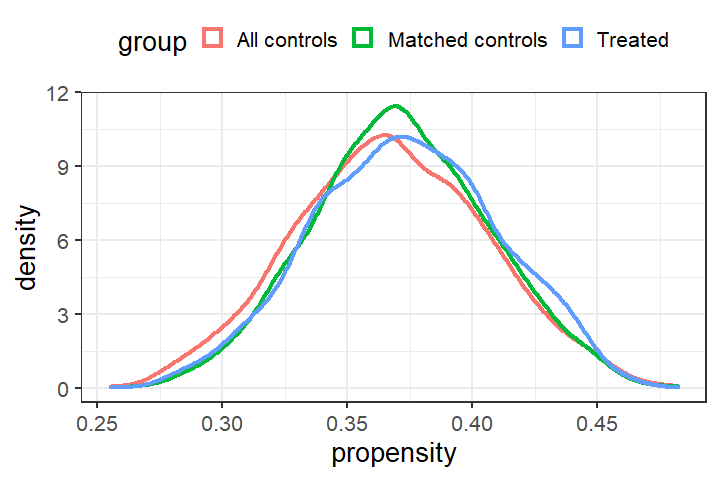print(tb_ex3_1) #> Z = 1 Z = 0 (Bef) Std. Diff (Bef) Z = 0 (Aft) Std. Diff (Aft) #> female 0.580 0.558 0.0321 0.586 -0.00891 #> black 0.191 0.185 0.0106 0.187 0.00645 #> bytest 51.884 53.040 -0.0976 52.551 -0.05629 #> fincome 21630.125 23439.164 -0.0722 21230.392 0.01595 #> dadeduc 1.102 1.171 -0.0409 1.102 0.00000 #> momeduc 0.935 0.996 -0.0375 0.935 0.00000 #> propensity 0.373 0.367 0.1146 0.371 0.03616 tb_ex3_2 = check_balance(Z, matching_output_ex3_2, cov_list = c('female', 'black', 'bytest', 'fincome', 'dadeduc', 'momeduc', 'propensity'), plot_propens = TRUE, propens = propensity)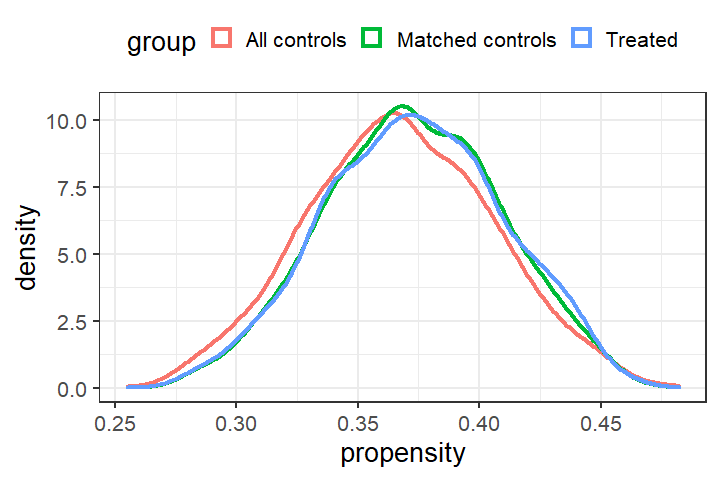print(tb_ex3_2) #> Z = 1 Z = 0 (Bef) Std. Diff (Bef) Z = 0 (Aft) Std. Diff (Aft) #> female 0.580 0.558 0.0321 0.580 0.000000 #> black 0.191 0.185 0.0106 0.191 0.000000 #> bytest 51.884 53.040 -0.0976 52.029 -0.012234 #> fincome 21630.125 23439.164 -0.0722 21640.374 -0.000409 #> dadeduc 1.102 1.171 -0.0409 1.102 0.000000 #> momeduc 0.935 0.996 -0.0375 0.935 0.000000 #> propensity 0.373 0.367 0.1146 0.373 0.010401 ### Example IV: Optimal matching with fine balance or near-fine balance We illustrate how to perform an optimal matching with fine balance and exact matching. Exact macthing is a constraint on who is matched to whom and hence implemented using the left network. We build a Mahalanobis-distance-based distance list subject to a propensity score caliper and exact matching constraints on female and black; see dist_list_1. On the other hand, fine balance is concerned about the marginal distribution, not who gets to matched to whom, and hence is implemented using the right network. On the right, we build a distance list that uses a 0/1 distance on variables father’s education and mother’s education, so that the joint distribution of father’s education and mother’s education are to be finely balanced; see dist_list_2. One can check the resulting match has exactly matched on female and black, and finely balanced father’s and mother’s education. dist_list_1 = create_list_from_scratch(Z = Z, X = X, exact = c('female', 'black'), p = propensity, caliper_low = 0.15, method = 'maha') dist_list_2 = create_list_from_scratch(Z = Z, X = X[, c('dadeduc', 'momeduc')], method = '0/1') matching_output_ex4 = match_2C_list(Z, dt_Rouse, dist_list_1, dist_list_2, lambda = 1000) tb_ex4 = check_balance(Z, matching_output_ex4, cov_list = c('female', 'black', 'bytest', 'fincome', 'dadeduc', 'momeduc', 'propensity'), plot_propens = TRUE, propens = propensity)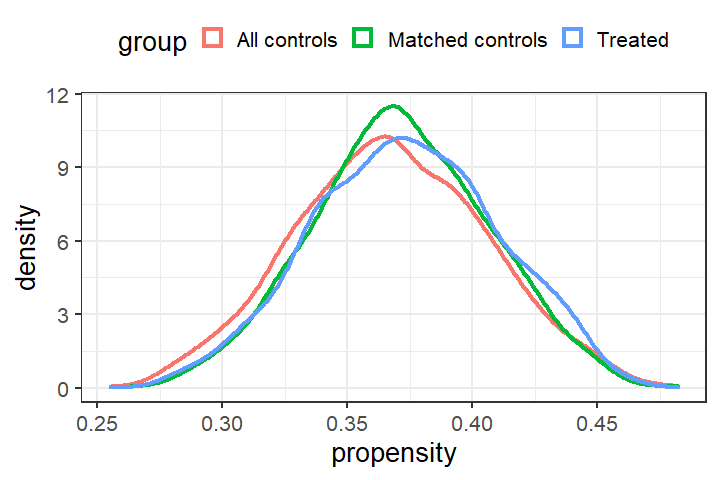print(tb_ex4) #> Z = 1 Z = 0 (Bef) Std. Diff (Bef) Z = 0 (Aft) Std. Diff (Aft) #> female 0.580 0.558 0.0321 0.580 0.00000 #> black 0.191 0.185 0.0106 0.191 0.00000 #> bytest 51.884 53.040 -0.0976 52.416 -0.04493 #> fincome 21630.125 23439.164 -0.0722 21646.168 -0.00064 #> dadeduc 1.102 1.171 -0.0409 1.102 0.00000 #> momeduc 0.935 0.996 -0.0375 0.935 0.00000 #> propensity 0.373 0.367 0.1146 0.371 0.03752 ### Example V: Force certain controls in the final matched cohort To force certain controls in the matched cohort, researchers may further apply the function force_control to the constructed distance lists. Below, we update the dist_list_1 and dist_list_2 from Example IV, and force the first 50 controls in the final matched cohorts, while still retain exact matching on female and black, and near-fine balance on father’s education and mother’s education.  # The first 50 controls must be included include_vec = c(rep(1, 50), rep(0, n_c - 50)) # dist_list_1 and dist_list_2 are from example 4 above dist_list_1_update = force_control(dist_list_1, Z = Z, include = include_vec) dist_list_2_update = force_control(dist_list_2, Z = Z, include = include_vec) matching_output_ex5 = match_2C_list(Z, dt_Rouse, dist_list_1_update, dist_list_2_update, lambda = 1000) tb_ex5 = check_balance(Z, matching_output_ex5, cov_list = c('female', 'black', 'bytest', 'fincome', 'dadeduc', 'momeduc', 'propensity'), plot_propens = TRUE, propens = propensity)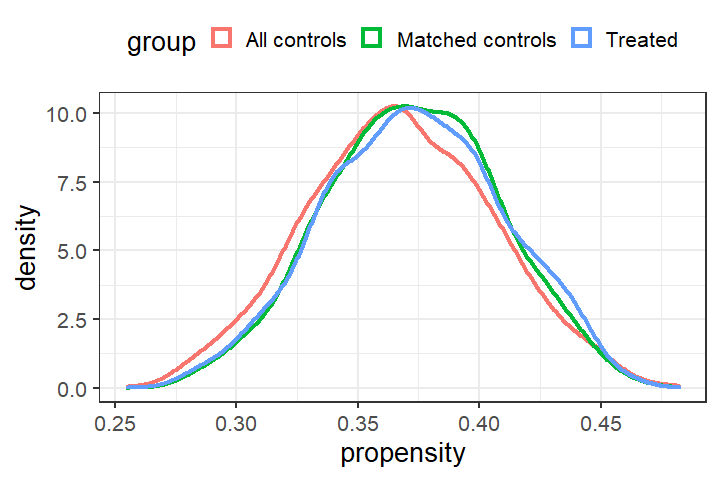print(tb_ex5) #> Z = 1 Z = 0 (Bef) Std. Diff (Bef) Z = 0 (Aft) Std. Diff (Aft) #> female 0.580 0.558 0.0321 0.580 0.00000 #> black 0.191 0.185 0.0106 0.191 0.00000 #> bytest 51.884 53.040 -0.0976 52.123 -0.02023 #> fincome 21630.125 23439.164 -0.0722 21434.046 0.00782 #> dadeduc 1.102 1.171 -0.0409 1.102 0.00000 #> momeduc 0.935 0.996 -0.0375 0.935 0.00000 #> propensity 0.373 0.367 0.1146 0.373 0.01354 ### Example VI: Recover the match_2C default match using match_2C_list As the last example, we show how to construct the default matching in the match_2C main function by constructing appropriate distance lists for the left and right networks. We construct the left network using Mahalanobis distance subject to the propensity score caliper and the exact matching constraints. This is dist_list_left. The right network has two parts. We first consturct a distance list based on the L1 distance of the propensity score. This distance list serves to balance the marginal distribution of the propensity score. This is dist_list_right_pscore. We then construct a distance list based on 0/1 distance on the variables to be finely balanced; see Example IV. This is dist_list_right_fb. We then combined these two distance lists and call it dist_list_right, and we use a penalty 100 to prioritize the fine balance constraint over balancing the propensity score. Optionally, one can use force_control function to force including certain controls; see Example V. Lastly, we use function match_2C_list to solve the resulting optimization problem, where we use a penalty lambda = 100 to priority the right network over the left. One can check that the match below exactly matches on black, finely balances father’s education, maximally balances the propensity score distributions in two groups, and uses the first 50 controls. # Construct distance list on the left: Maha subject to pscore caliper dist_list_left = create_list_from_scratch(Z = Z, X = X, exact = c('black'), p = propensity, caliper_low = 0.2, method = 'maha') # Construct distance list on the right: L1 distance on the pscore plus fine balance dist_list_right_pscore = create_list_from_scratch(Z = Z, X = propensity, p = propensity, caliper_low = 1, method = 'L1') dist_list_right_fb = create_list_from_scratch(Z = Z, X = X[, c('dadeduc')], p = propensity, caliper_low = 1, method = '0/1') dist_list_right = dist_list_right_pscore dist_list_right$d = dist_list_right$d + 100*dist_list_right_fb$d

# The first 50 controls must be included
include_vec = c(rep(1, 50), rep(0, n_c - 50))

# dist_list_1 and dist_list_2 are from example 4 above
dist_list_left_update = force_control(dist_list_left, Z = Z, include = include_vec)
dist_list_right_update = force_control(dist_list_right, Z = Z, include = include_vec)

matching_output_ex6 = match_2C_list(Z = Z, dataset = dt_Rouse,
dist_list_1 = dist_list_left_update,
dist_list_2 = dist_list_right_update,
lambda = 100)

tb_ex6 = check_balance(Z, matching_output_ex6,
cov_list = c('female', 'black', 'bytest', 'fincome', 'dadeduc', 'momeduc', 'propensity'),
plot_propens = TRUE, propens = propensity)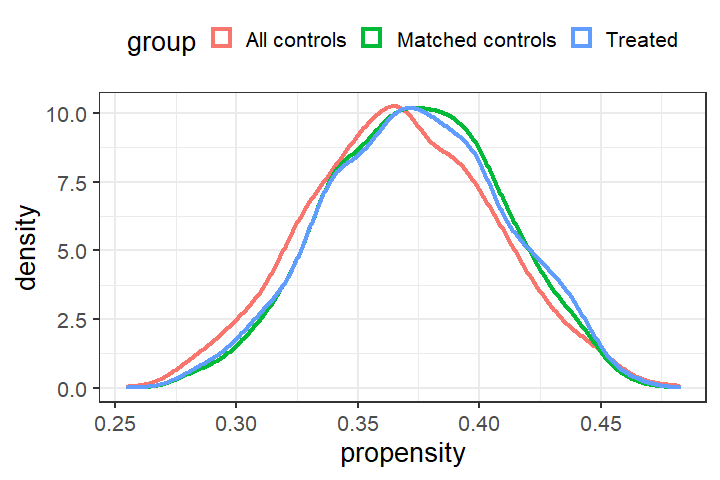print(tb_ex6)
#>                Z = 1 Z = 0 (Bef) Std. Diff (Bef) Z = 0 (Aft) Std. Diff (Aft)
#> female         0.580       0.558          0.0321       0.586       -0.007635
#> black          0.191       0.185          0.0106       0.191        0.000000
#> bytest        51.884      53.040         -0.0976      52.048       -0.013890
#> fincome    21630.125   23439.164         -0.0722   21188.057        0.017637
#> dadeduc        1.102       1.171         -0.0409       1.102        0.000000
#> momeduc        0.935       0.996         -0.0375       0.888        0.029094
#> propensity     0.373       0.367          0.1146       0.373        0.000773